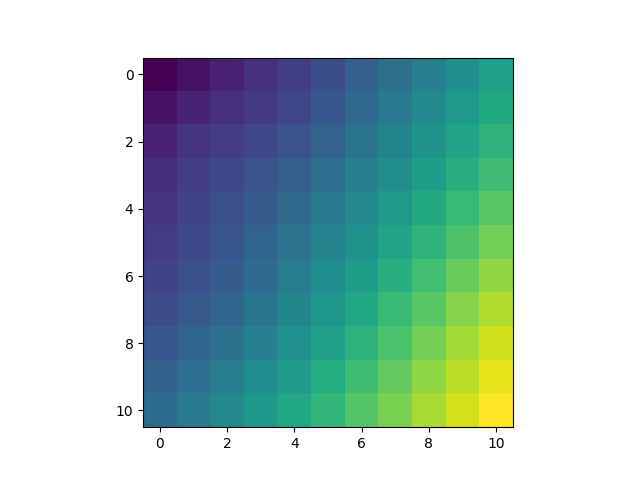# Universal Kriging Example

In this example we apply a regional linear trend to the kriging system.

```import matplotlib.pyplot as plt
import numpy as np

from pykrige.uk import UniversalKriging

data = np.array(
[
[0.3, 1.2, 0.47],
[1.9, 0.6, 0.56],
[1.1, 3.2, 0.74],
[3.3, 4.4, 1.47],
[4.7, 3.8, 1.74],
]
)

gridx = np.arange(0.0, 5.5, 0.5)
gridy = np.arange(0.0, 5.5, 0.5)
```

Create the universal kriging object. Required inputs are the X-coordinates of the data points, the Y-coordinates of the data points, and the Z-values of the data points. Variogram is handled as in the ordinary kriging case. drift_terms is a list of the drift terms to include; currently supported terms are ‘regional_linear’, ‘point_log’, and ‘external_Z’. Refer to UniversalKriging.__doc__ for more information.

```UK = UniversalKriging(
data[:, 0],
data[:, 1],
data[:, 2],
variogram_model="linear",
drift_terms=["regional_linear"],
)
```

Creates the kriged grid and the variance grid. Allows for kriging on a rectangular grid of points, on a masked rectangular grid of points, or with arbitrary points. (See UniversalKriging.__doc__ for more information.)

```z, ss = UK.execute("grid", gridx, gridy)
plt.imshow(z)
plt.show()
```Total running time of the script: ( 0 minutes 0.151 seconds)

Gallery generated by Sphinx-Gallery